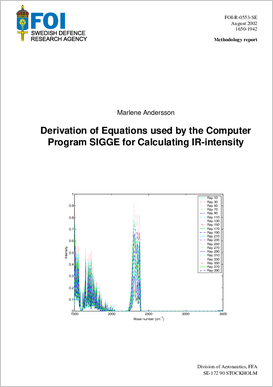# Derivation of equations used by the computer program SIGGE for calculating IR-intensityAuthors:

Publish date: 2002-01-01

Report number: FOI-R--0553--SE

Pages: 37

Written in: English

## Abstract

To be able to predict the IR-signature of an aircraft for example, the heat radiated, in form of IR-radiation, from the aircraft has to be calculated. The module based code SIGGE, for calculating the IR-signature, is under development. This code uses a number of equations to calculate the IR-intensity. This report describes how these equations are derived together with a brief discussion about radiative heat transfer.